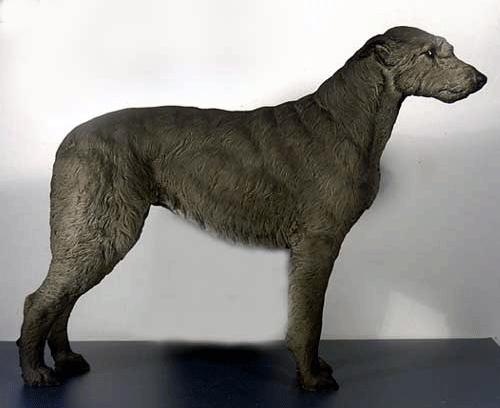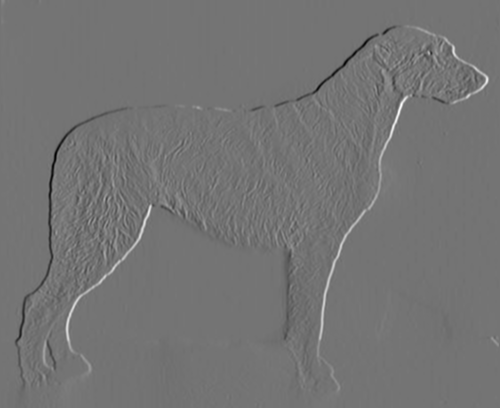Pipeline examples¶

:
from easycv import Image, Pipeline
from easycv.transforms import Gradient, Blur, Rotate

For this example we will load an image using random().

:
img = Image.random()
img
:Creating pipeline¶

To create a pipeline we simply call it’s constructor with a list of transforms.

:
pipeline
:
Pipeline (test) with 2 transforms
2: Blur (method=gaussian, size=auto, sigma=0, sigma_color=75, sigma_space=75, truncate=4)
:
img.apply(pipeline)
:We can also create a pipeline with another pipeline inside

:
pipeline = Pipeline([pipeline, Rotate(degrees=20)],name="test")
pipeline
:
Pipeline (test) with 3 transforms
1: Pipeline (test) with 2 transforms
|    1: Gradient (axis=x, method=sobel, size=5)
|    2: Blur (method=gaussian, size=auto, sigma=0, sigma_color=75, sigma_space=75, truncate=4)
2: Rotate (degrees=20, scale=1, center=auto, original=True)

And it’s possible to add a transform to an existing pipeline

:
pipeline
:
Pipeline (test) with 4 transforms
1: Pipeline (test) with 2 transforms
|    1: Gradient (axis=x, method=sobel, size=5)
|    2: Blur (method=gaussian, size=auto, sigma=0, sigma_color=75, sigma_space=75, truncate=4)
2: Rotate (degrees=20, scale=1, center=auto, original=True)
3: Rotate (degrees=-40, scale=1, center=auto, original=True)

Number of transforms¶

You can check the number of transforms in a pipeline. This is the number of Transforms so nested pipelines count as their internal number of Transforms

:
pipeline.num_transforms()
:
4

Save¶

If you want you can save a pipeline for future use or sharing

:
pipeline.save(filename="pipeline")